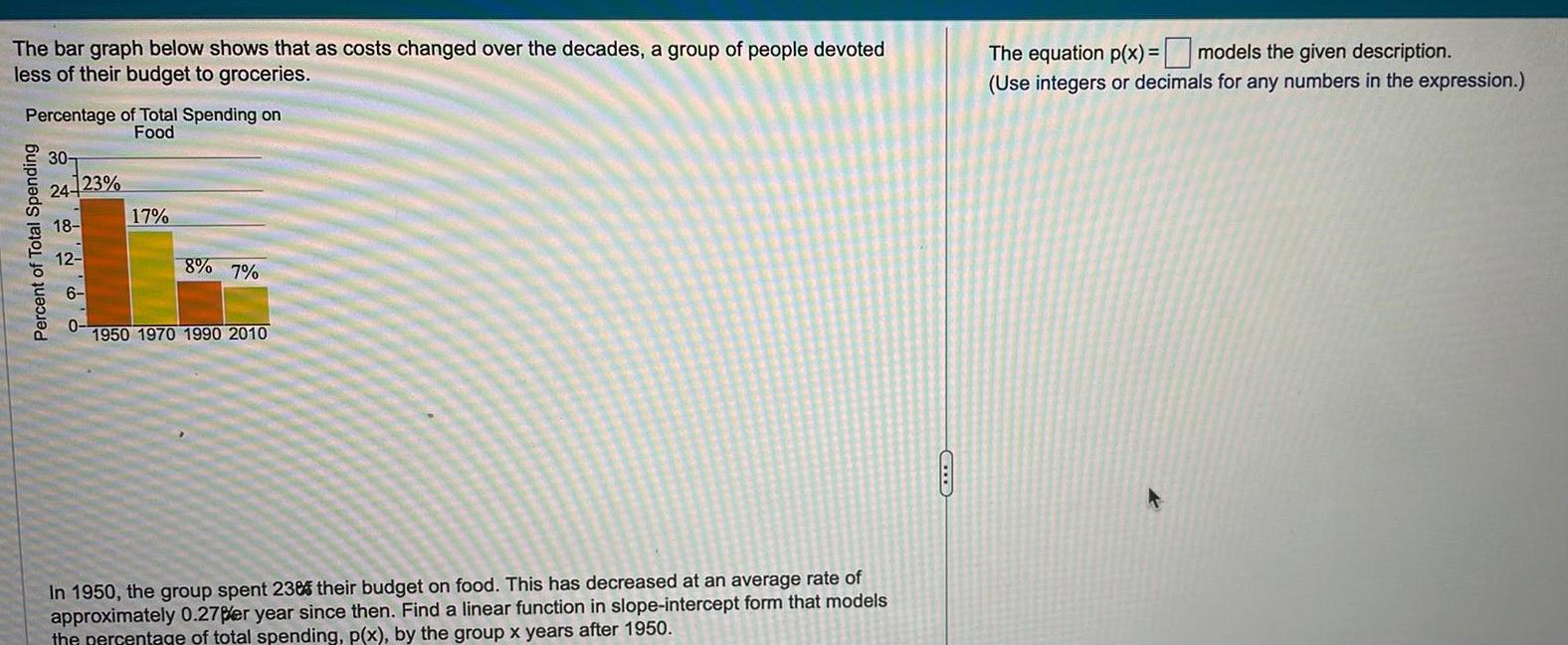Question:

# The bar graph below shows that as costs changed over the

Last updated: 9/18/2023The bar graph below shows that as costs changed over the decades a group of people devoted less of their budget to groceries Percentage of Total Spending on Food Percent of Total Spending 30 24 23 18 12 17 8 7 1950 1970 1990 2010 In 1950 the group spent 238 their budget on food This has decreased at an average rate of approximately 0 27per year since then Find a linear function in slope intercept form that models the percentage of total spending p x by the group x years after 1950 The equation p x models the given description Use integers or decimals for any numbers in the expression